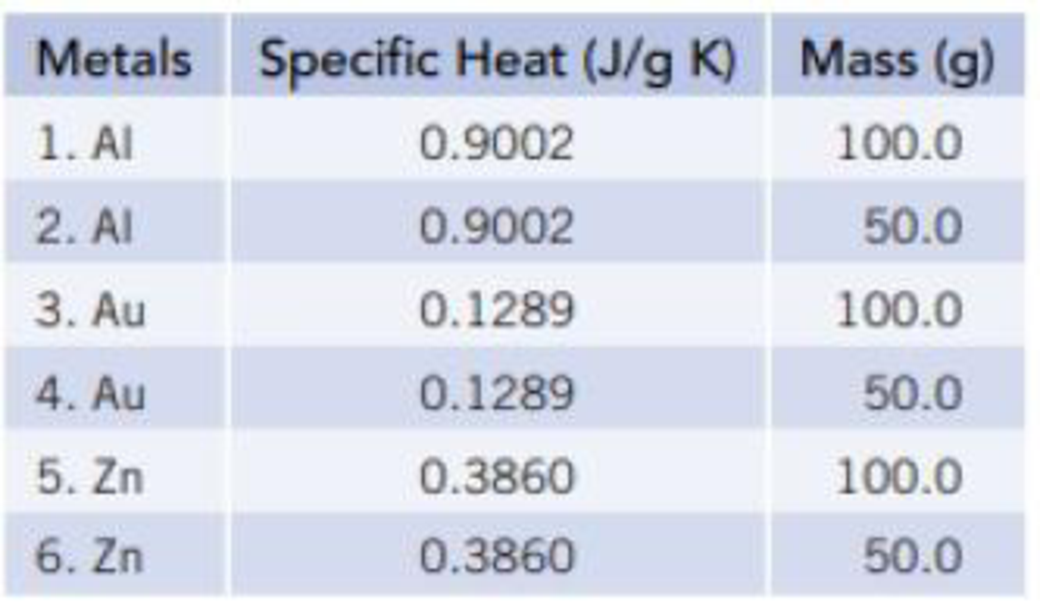Chapter 5, Problem 117SCQ

Chapter
Section
Textbook Problem

You have the six pieces of metal listed below, plus a beaker of water containing 3.00 × 102 g of water. The water temperature is 21.00 °C.(a) In your first experiment you select one piece of metal and heat it to 100 °C, and then select a second piece of metal and cool it to −10 °C. Both pieces of metal are then placed in the beaker of water and the temperatures equilibrated. You want to select two pieces of metal to use, such that the final temperature of the water is as high as possible. What piece of metal will you heat? What piece of metal will you cool? What is the final temperature of the water? (b) The second experiment is done in the same way as the first. However, your goal now is to cause the temperature to change the least, that is, the final temperature should be as near to 21.00 °C as possible. What piece of metal will you heat? What piece of metal will you cool? What is the final temperature of the water?

(a)

Interpretation Introduction

Interpretation:

The piece of the metal which is heated and cooled and the final temperature of the water have to be determined

Concept Introduction:

The standard enthalpy of formation is the enthalpy change for the formation of 1mol of the compound directly from its component elements in their standard states.

Enthalpy change for the reaction ΔrH0ΣnΔfH0(products)-ΣnΔfH0(reactants)

Explanation

To heat at maximum, the metal with highest specific heat capacity is good. So aluminum is preferred.

To cool the metal to its minimum, the metal with lowest specific heat capacity is good. So gold is preferred

Assume the heat loss by the warm metal = Heat gain by the cool metal and water.

i.e. the -heatloss=heatgain

-[100g×0.9002J/gK(T-373K)] =[50g×0.1289J/gK(T-263)]+[300g×4

(b)

Interpretation Introduction

Interpretation:

The change in internal energy in the system has to be calculated.

Concept Introduction:

Standard enthalpy of the reaction,ΔrHo, is the change in enthalpy that happens when matter is transformed by a given chemical reaction, when all reactants and products are in their standard states.

Enthalpy change for the reaction ΔrH0=ΣnΔfH0(products)-ΣnΔfH0(reactants)

Still sussing out bartleby?

Check out a sample textbook solution.

See a sample solution

The Solution to Your Study Problems

Bartleby provides explanations to thousands of textbook problems written by our experts, many with advanced degrees!

Get Started